• 小明种苹果 if __name__ == "__main__": s = list(map(int,input().split())) n = s#表示苹果树的个数 m = s#表示蔬果操作的轮数 T = 0 p = 0 k = -1 for i in range(1,n+1): cur = list(map(int,...
小明种苹果if __name__ == "__main__":
s = list(map(int,input().split()))
n = s#表示苹果树的个数
m = s#表示蔬果操作的轮数
T = 0
p = 0
k = -1
for i in range(1,n+1):
cur = list(map(int,input().split()))
cur_sum = abs(sum(cur[1:]))
T+=sum(cur)
if p<cur_sum:
p = cur_sum
k = i
print(T,k,p)




展开全文python
• 小明种苹果 时间限制： 2.0s 内存限制： 512.0MB 问题描述： #include <iostream> using namespace std; int main() { int i,n,m,a,b[20...
试题编号：
201909-1
试题名称：
小明种苹果
时间限制：
2.0s
内存限制：
512.0MB
问题描述：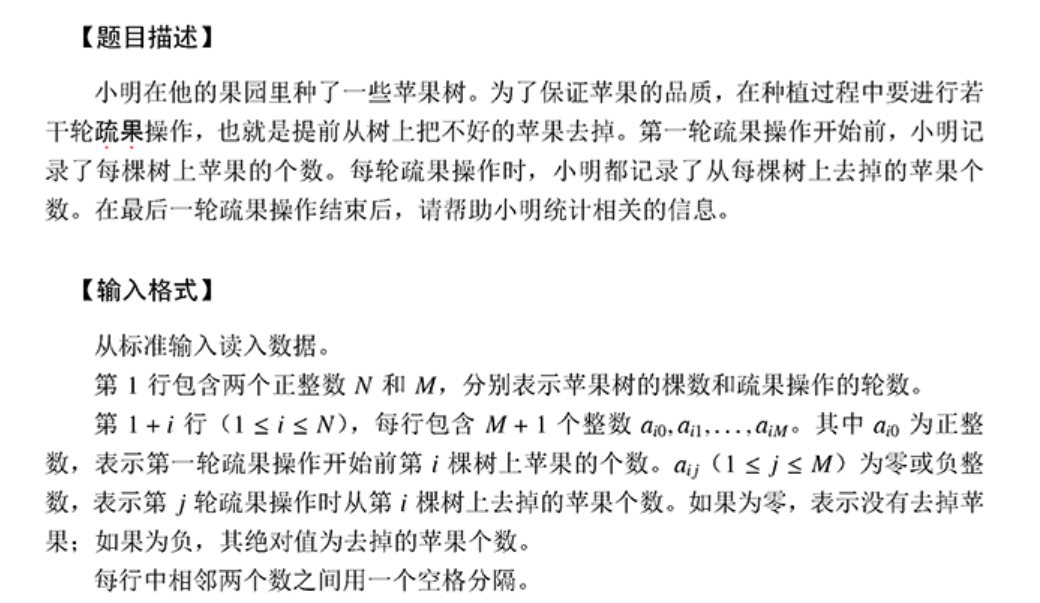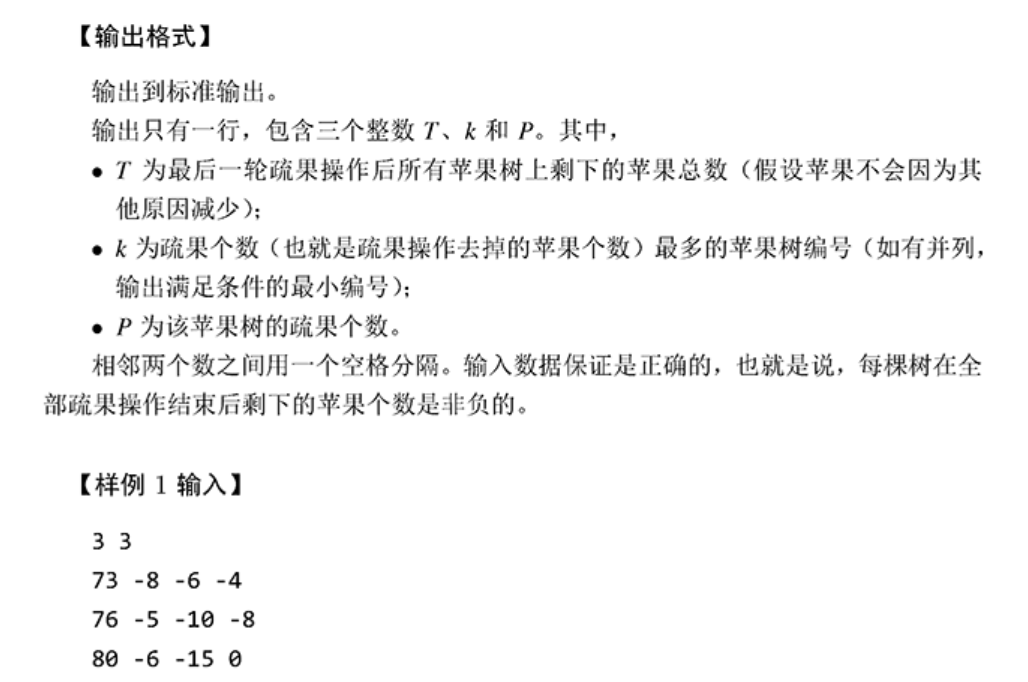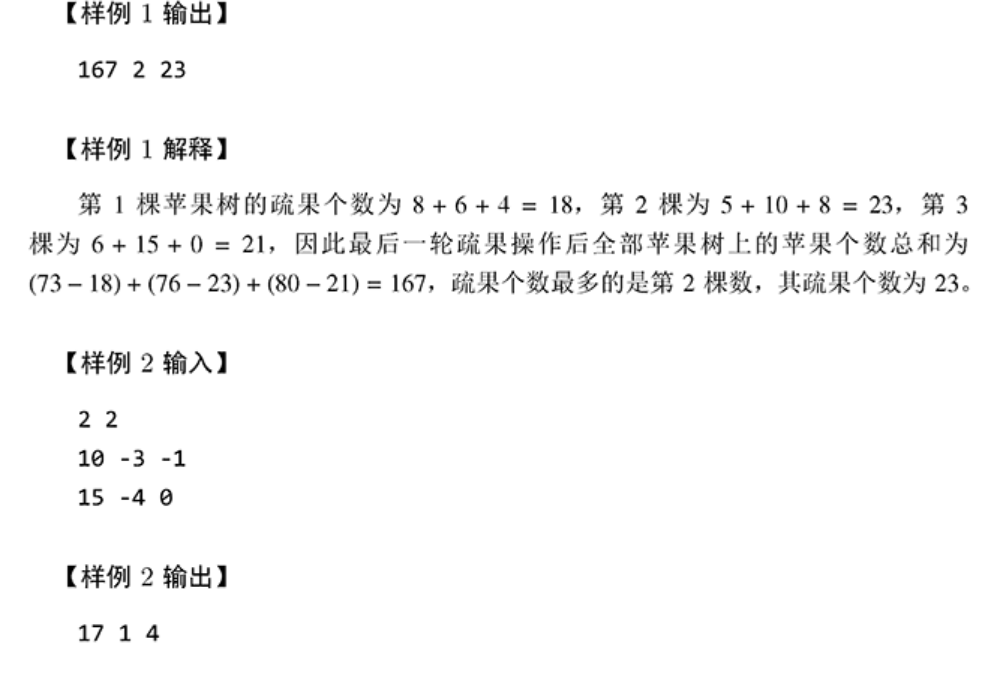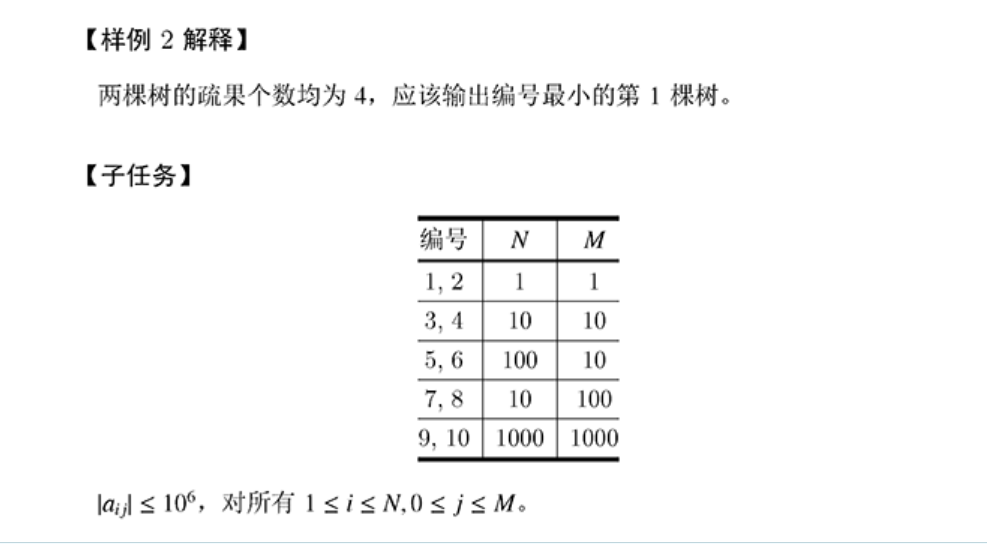#include <iostream>

using namespace std;

int main()
{
int i,n,m,a,b;
cin>>n>>m;
for(i=1; i<=n; i++)
{
cin>>a[i];
b[i]=a[i];
for(int j=1; j<=m; j++)
{
int x;
cin>>x;
if(x<=0) x=x*(-1);
b[i]=b[i]-x;
}
}
int t=0,k=0,p=1;
for(i=1; i<=n; i++)
{
t+=b[i];
if((a[i]-b[i])>k)k=a[i]-b[i],p=i;

}
cout<<t<<" "<<p<<" "<<k<<endl;
return 0;
}



展开全文• 想必大家是在这个题上出来问题才来找解答的，题目是什么大家应该都清楚，就不再叙述了。 这个题没什么难度，主要是在一个细节上要注意： 千万不要在函数里定义二维数组 第9，10个数据，当N和M同时为1000时，二维数组...
想必大家是在这个题上出来问题才来找解答的，题目是什么大家应该都清楚，就不再叙述了。
这个题没什么难度，主要是在一个细节上要注意：
千万不要在函数里定义二维数组
第9，10个数据，当N和M同时为1000时，二维数组是不能承受的。
一个1000X1000的int数组大概是4M左右，定义这样的数组就会报错。
这里提供的解决方案是定义一维数组，因为输入的数据只用了一次，所以覆盖掉也没什么问题。
要讲的就这么多，有什么问题可以提问。
下面是代码
#include<iostream>
using namespace std;
int main()
{    int n,m;
cin>>n>>m;
const int a=n,b=m+1;
int x[b];
int count=0,max=0,k=0;
for(int i=0;i<a;i++)
{
int c=0;
for(int j=0;j<b;j++)
{
cin>>x[j];
if(j)
c-=x[j];
}
count+=x-c;
if(c>max)
{
max=c;
k=i+1;
}
}
cout<<count<<" "<<k<<" "<<max<<'\n';
return 0;
}


展开全文• ccf小明种苹果 （续） #ccf小明种苹果 （续） n = int(input()) a, d = [], [] for i in range(n): a.append(list(map(int, input().split()))) t, p, k = 0, 0, 0 for i in range(n): a[i].append(0)...
ccf小明种苹果 （续）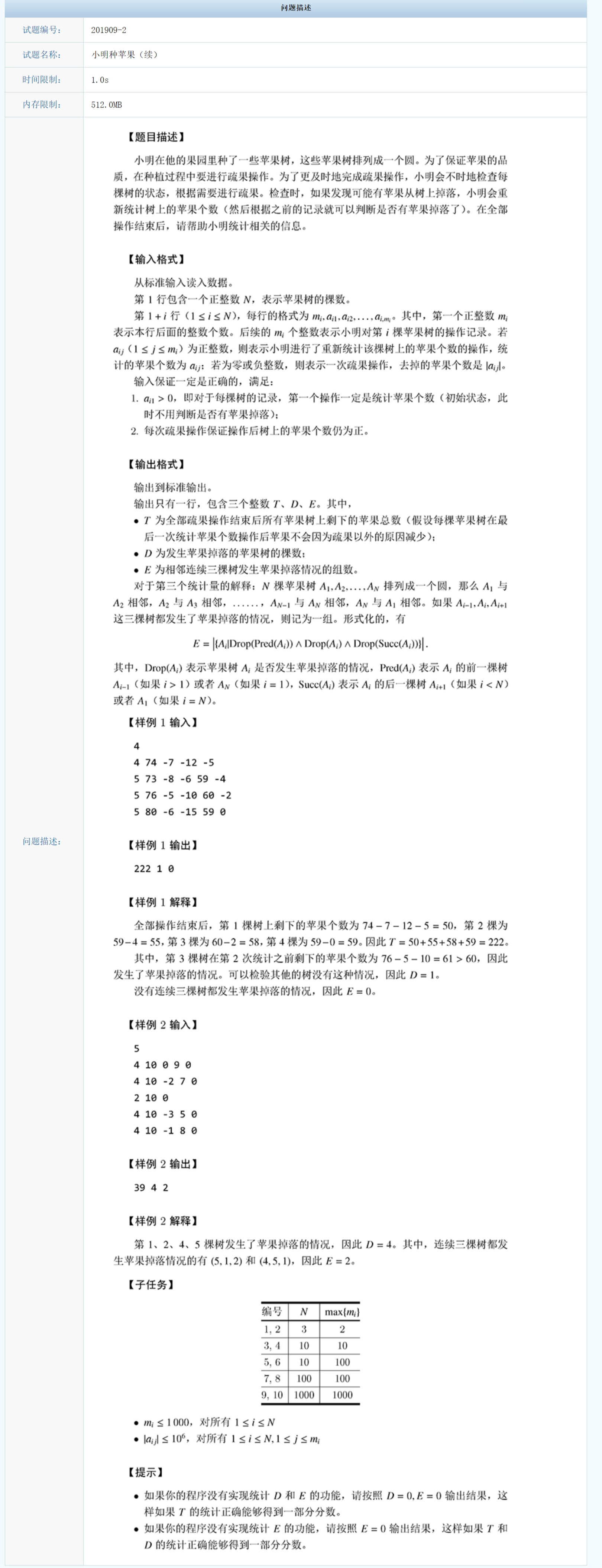#ccf小明种苹果 （续）
n = int(input())
a, d = [], []
for i in range(n):
a.append(list(map(int, input().split())))

t, p, k = 0, 0, 0

for i in range(n):
a[i].append(0)
temp = a[i]
if a[i] >= 1:
for j in range(2, a[i]+1):
if a[i][j] <= 0:
temp += a[i][j]
else:
#if temp > a[i][j] and len(d)>0 and d[-1]!=i: #len(d)>0导致第一次加不上
if temp > a[i][j] and (len(d) == 0 or (len(d) > 0 and d[-1] != i)):
d.append(i)   #一棵树掉几次的情况？？？
temp = a[i][j]
a[i][-1] = temp

for i in range(n):
t += a[i][-1]

e = 0

for i in range(len(d) - 2):
if d[i + 1] == d[i]+1 and d[i + 2] == d[i + 1] + 1: e += 1

if len(d) >= 3:
if d == 0 and d == 1 and d[-1] == n - 1:
e += 1

if d == 0 and d[-2] == n - 2 and d[-1] == n - 1:
e += 1

print(t, len(d), e)




展开全文python
• ccf小明种苹果（续） 这个题目难度不大，可能有问题就是连续三个树为苹果掉落，我着重在这个部分讲一下 #include<bits/stdc++.h> using namespace std; struct node{ int flag; int num; int sum; }; ...
• 小明种苹果（续） if __name__ == "__main__": n = int(input()) T = 0 D = 0 E = 0 flag = [0 for _ in range(n)] for i in range(n): s = list(map(int,input().split())) m = s cur = s for ...python
• 小明种苹果20190901题目分析源码 题目 Case 1： 输入： 3 3 73 -8 -6 -4 76 -5 -10 -8 80 -6 -15 0 输出： 167 2 23 解释： 第1棵苹果树的蔬果个数为8+6+4=18，第2棵为5+10+8=23，第3棵为6+15+0=21，因此最后...算法
• #include<stdio.h> int a; int b={0}; int main(){ int N,M,i,j,T,k,P=0; scanf("%d %d",&N,&M); for(i=0;i<N;i++){ for(j=0;j<M+1;j++){ ...a[i][j...
• N,M = map(int,input().split()) total = [] dictionary = {} for i in range(N): temp = list(map(int,input().split())) total.append(sum(temp)) dictionary[i+1] =abs(sum(temp[1:])) maxval = 0 ...
• 小明种苹果（续）20190902题目分析源码 题目 Case 1: 输入： 4 4 74 -7 -12 -5 5 73 -8 -6 59 -4 5 76 -5 -10 60 -2 5 80 -6 -15 59 0 输出： 222 1 0 解释： Case 2: 输入： 5 4 10 0 9 0 4 10 -2 7 0 2 10 ...算法
• [](http://118.190.20.162/view.page?gpid=T94 "") #include using namespace std; int main(){ int n,m,k=0; cin>>n>>m; int a[n][m+3]; for(int i=0;i;i++){ int sum=0;...}c++
• 思路 简单模拟即可 #include <iostream> using namespace std; const int maxn = 1005; int now, flag[maxn], N, m, T, D, E; int main() { scanf("%d", &N); for(int i = 0;... for(int j = ...Java
• 原题 官网放图真受不了，案例一个一个敲的 测试样例 """ 5 4 10 0 9 0 4 10 -2 7 0 2 10 0 4 10 -3 5 0 4 10 -1 8 0 4 4 74 -7 -12 -5 5 73 -8 -6 59 -4 5 76 -5 -10 60 -2 5 80 -6 -15 59 0 ...用temp保存...
• i 再刷CCF的题目，这道题是小模拟，我自己刷题很少，也是做了2个小时才做出来，速度太慢，加油，希望可以考过200分 下面是我用python写的 n = list(map(int, input().split())) M = [] Q = [] while n != 0: s = ...C++
• #include <iostream> using namespace std; int a; int b; int main(){ int N;...//数组c[]用来记录苹果自动掉落的树的号码 //c=-1为了判断当下标为0的树是否有自然掉落的 fo...c++ 算法
• 1.小明种苹果 题目描述 小明在他的果园里种了一些苹果树。为了保证苹果的品质，在种植过程中要进行若干轮疏果操作，也就是提前从树上把不好的苹果去掉。第一轮疏果操作开始前，小明记录了每棵树上苹果的个数。每轮...
• 小明在他的果园里了一-些苹果树。为了保证苹果的品质，在种植过程中要进行若 干轮疏果操作，也就是提前从树上把不好的苹果去掉。第- ~轮疏果操作开始前，小明记 录了每棵树上苹果的个数。每轮疏果操作时，小明都...
• 小明种苹果 思路 两大坑点： 一颗苹果树可能进行多次疏果。只要当前苹果树上苹果的值大于输入的那个正数的值，就表示发生了落果。 当苹果树只有三棵时，且均有落果，则E为3，不是1。斯认为这个很不科学，就应该是1。...
• 201909-（2）小明种苹果续 #include <iostream> #define _for(i,a,b) for(int i=a;i<b;i++) using namespace std; int flag; //连续掉落flag int d; //掉落flag int main(){ int T=0,D=0,E=...c++
• 思路：将初始的树上苹果数和疏过操作过程分别存在两个数组中。分别对两个数组操作，求出每棵树上的疏果数和疏果后的苹果总数。扫描每棵树上的疏果数的数组，标记出最多的疏果的树。 代码实现： #include<...
• CCF 201902 小明种苹果（续） package cf; import java.util.Scanner; public class Mainapplexu { public static void main(String[] args) { Scanner sc = new Scanner(System.in); int n = sc.nextInt()......